# NCERT Solution: Triangle and Its Properties- 1 Notes | Study Mathematics (Maths) Class 7 - Class 7

## Class 7: NCERT Solution: Triangle and Its Properties- 1 Notes | Study Mathematics (Maths) Class 7 - Class 7

The document NCERT Solution: Triangle and Its Properties- 1 Notes | Study Mathematics (Maths) Class 7 - Class 7 is a part of the Class 7 Course Mathematics (Maths) Class 7.
All you need of Class 7 at this link: Class 7

Exercise 6.1

Q1. In APQR, D is the mid-point of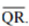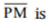____________
PD is_____________
Is QM = MR?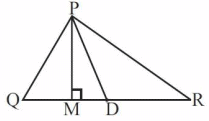Ans: Given: QD = DR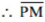is altitude.
PD is median.
No, QM (eq) MR as D is the mid-point of QR.

Q2. Draw rough sketches for the following:

(a) In ΔABC, BE is a median.
Ans: Here, BE is a median in ΔABC and AE = EC.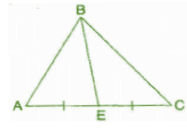(b) In ΔPQR, PQ and PR are altitudes of the triangle.
Ans: Here, PQ and PR are the altitudes of the ΔPQR and RP ⊥ QP.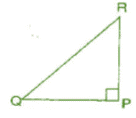(c) In ΔXYZ, YL is an altitude in the exterior of the triangle.
Ans: YL is an altitude in the exterior of ΔXYZ.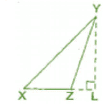Q3. Verify by drawing a diagram if the median and altitude of a isosceles triangle can be same.
Ans: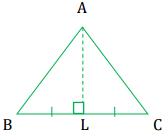Isosceles triangle means any two sides are same.
Take ΔABC and draw the median when AB = AC.
AL is the median and altitude of the given triangle.

Exercise 6.2

Q1. Find the value of the unknown exterior angle x in the following diagrams: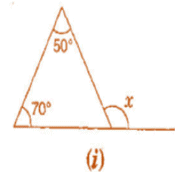Ans: We Know That,
An exterior angle of a triangle is equal to the sum of its interior opposite angles.
= x = 50° + 70°
= x = 120°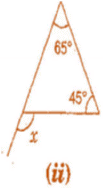Ans: We Know That,
An exterior angle of a triangle is equal to the sum of its interior opposite angles.
= x = 65° + 45°
= x = 110°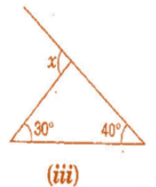Ans: We Know That, An exterior angle of a triangle is equal to the sum of its interior opposite angles.
= x = 30° + 40°
= x = 70°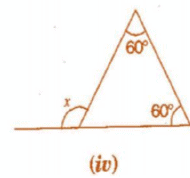Ans: We Know That, An exterior angle of a triangle is equal to the sum of its interior opposite angles.
= x = 60° + 60°
= x = 120°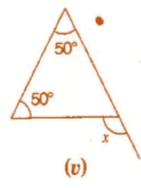Ans: We Know That,
An exterior angle of a triangle is equal to the sum of its interior opposite angles.
= x = 50° + 50°
= x = 100°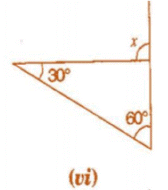Ans: We Know That,
An exterior angle of a triangle is equal to the sum of its interior opposite angles.
= x = 30° + 60°
= x = 90°

Q2. Find the value of the unknown interior angle x in the following figures: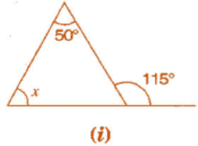Ans: We Know That,
An exterior angle of a triangle is equal to the sum of its interior opposite angles.
= x + 50° = 115°
By transposing 50° from LHS to RHS it becomes – 50°
= x = 115° – 50°
= x = 65°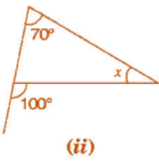Ans: We Know That,
An exterior angle of a triangle is equal to the sum of its interior opposite angles.
= 70o + x = 100°
By transposing 70° from LHS to RHS it becomes – 70°
= x = 100° – 70°
= x = 30°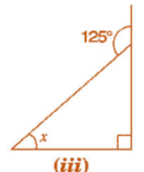Ans: We Know That,
An exterior angle of a triangle is equal to the sum of its interior opposite angles.
The given triangle is a right angled triangle. So the angle opposite to the x is 90°.
= x + 90° = 125°
By transposing 90° from LHS to RHS it becomes – 90°
= x = 125° – 90°
= x = 35°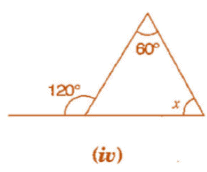Ans: We Know That,
An exterior angle of a triangle is equal to the sum of its interior opposite angles.
= x + 60° = 120°
By transposing 60° from LHS to RHS it becomes – 60°
= x = 120° – 60°
= x = 60°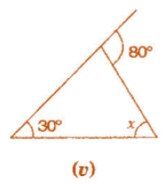Ans: We Know That,
An exterior angle of a triangle is equal to the sum of its interior opposite angles.
The given triangle is a right angled triangle. So the angle opposite to the x is 90°.
= x + 30° = 80°
By transposing 30° from LHS to RHS it becomes – 30°
= x = 80° – 30°
= x = 50°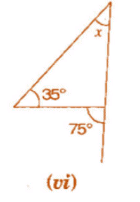Ans: We Know That,
An exterior angle of a triangle is equal to the sum of its interior opposite angles.
The given triangle is a right angled triangle. So the angle opposite to the x is 90°.
= x + 35° = 75°
By transposing 35° from LHS to RHS it becomes – 35°
= x = 75° – 35°
= x = 40°

Exercise 6.3

Q1. Find the value of unknown x in the following diagrams: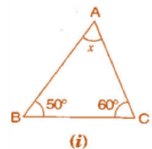Ans: In ΔABC,
∠ BAC + ∠ ACB + ∠ ABC = 180°  [ By angle sum property of a triangle]
⇒ x + 50°+ 60° = 180°
⇒  x  + 110° = 180°
⇒  x = 180°-110° = 70°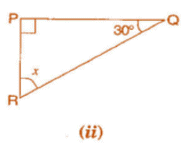Ans: In ΔPQR,
∠ RPQ + ∠ PQR + ∠ RPQ = 180° [By angle sum property of a triangle]
⇒  90° + 30°+ x = 180°
⇒  x + 120° = 180°
⇒   x= 180° - 120°= 60°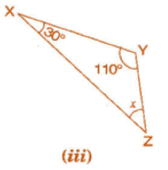Ans: In ΔXYZ,
∠ ZXY + ∠ XYZ + ∠ YZX = 180° [By angle sum property of a triangle]
⇒   30° + 110° + x = 180°
⇒  x + 140° = 180°
⇒ x = 180° - 140° = 40°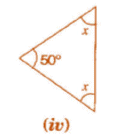Ans: In the given isosceles triangle,
x + x + 50° = 180°   [By angle sum property of a triangle]
⇒  2x + 50°= 180°
⇒  2x = 180°- 50°
⇒  2x = 130°
⇒ x = 130° / 2 = 65°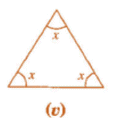Ans: In the given equilateral triangle,
x + x + x = 180° [By angle sum property of a triangle]
⇒ 3x = 180°
⇒ x = 180° / 3 = 60°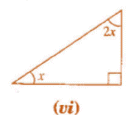Ans: In the given right angled triangle,
x + 2x + 90° = 180°    [By angle sum property of a triangle)
⇒  3x + 90° = 180°
⇒  3* = 180°- 90°
⇒ 3x = 90°
⇒ x = 90° / 3 = 30°

Q2. Find the values of the unknowns x and y in the following diagrams: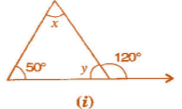Ans: 50° + x = 120°    [Exterior angle property of a Δ]
⇒   x = 120° - 50° = 70°
Now, 50° + x + y = 180°    [Angle sum property of a Δ]
⇒  50° + 70° + y = 180°
⇒  120° + y = 180°
⇒  y = 180° - 120° = 60°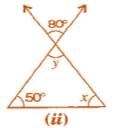Ans: y = 80° ..... (i)    [Vertically opposite angle]
Now, 50° + x + y = 180°    [Angle sum property of a Δ]
⇒  50° + 80°+y = 180°    [From equation (i)]
⇒ 130° + y = 180°
⇒ y = 180° - 130° = 50°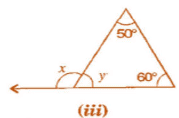Ans: 50°+ 60° = x    (Exterior angle property of a Δ]
x = 110°
Now 50° + 60°+ y = 180°    [Angle sum property of a Δ]
⇒  110° + y = 180°
⇒  y = 180° - 110°
⇒  y = 70°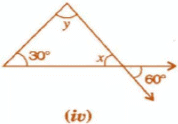Ans: x = 60° ..... (i) [Vertically opposite angle]
Now, 30° + x + y = 180°    [Angle sum property of a Δ ]
⇒  50° + 60° + y = 180°    [From equation (i)]
⇒ 90° + y = 180°
⇒  y = 180° - 90° = 90°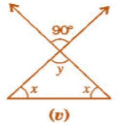Ans: y = 90°   ....(i)    [Vertically opposite angle]
Now, y + x + x = 180°    [Angle sum property of a Δ]
⇒  90° + 2x = 180°    [From equation (i)]
⇒ 2x = 180°- 90°
⇒  2x = 90°
⇒ x = 90° / 2 = 45°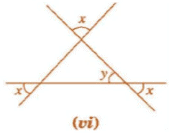Ans: x = y  ...(i)   [Vertically opposite angle]
Now, x + x + y = 180°    [Angle sum property of a Δ]
⇒ 2x + x = 180°    [From equation (i)]
⇒ 3x = 180°
⇒ x = 180° / 3 = 60°

The document NCERT Solution: Triangle and Its Properties- 1 Notes | Study Mathematics (Maths) Class 7 - Class 7 is a part of the Class 7 Course Mathematics (Maths) Class 7.
All you need of Class 7 at this link: Class 7Use Code STAYHOME200 and get INR 200 additional OFF

## Mathematics (Maths) Class 7

165 videos|252 docs|45 tests

Track your progress, build streaks, highlight & save important lessons and more!

,

,

,

,

,

,

,

,

,

,

,

,

,

,

,

,

,

,

,

,

,

;